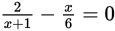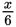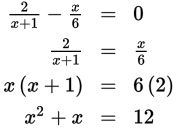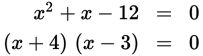# SAT Math Multiple Choice Question 898: Answer and Explanation

### Test Information

Question: 898

13.If x1 and x2 are valid solutions to the rational equation given, what is the sum of x1 + x2?

• A. -1
• B. 0
• C. 1
• D. 2

Explanation:

A

Difficulty: Medium

Category: Passport to Advanced Math / Exponents

Strategic Advice: When solving a rational equation (an equation with variables in the denominator) with exactly two terms, it is almost always quicker to set one term equal to the other (separate the terms on opposite sides of the = sign) and cross-multiply. You could also find a common denominator and go from there, but this usually takes more time.

Getting to the Answer: Addto both sides of the equation, and then cross-multiply:You now have a quadratic equation to solve. Start by subtracting 12 from both sides so that the equation equals 0. The answer choices are all integers, so this is a clue that you should be able to factor the equation:Set each factor equal to 0 and solve to find that x = -4 and x = 3. The sum of these values is -4 + 3 = -1, so (A) is correct.